# WBJEE Maths Test - 14

## 75 Questions MCQ Test WBJEE Sample Papers, Section Wise & Full Mock Tests | WBJEE Maths Test - 14

Description
Attempt WBJEE Maths Test - 14 | 75 questions in 120 minutes | Mock test for JEE preparation | Free important questions MCQ to study WBJEE Sample Papers, Section Wise & Full Mock Tests for JEE Exam | Download free PDF with solutions
QUESTION: 1

Solution:
QUESTION: 2

Solution:
QUESTION: 3

### In the expansion of (1+x)(2n+2) the maximum coefficient is :

Solution:
QUESTION: 4
The polar of a point P w.r.t a circle of radius a touching both x and y axis and lying in the first quadrant is x + 2y = 4a. The coordinates of P are
Solution:
QUESTION: 5

The limiting point of the system of co-axial circles x2+y2-6x-6y+4=0, x2+y2-2x-4y+3=0 is

Solution:
QUESTION: 6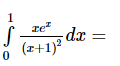Solution: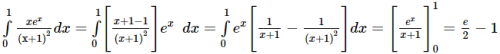QUESTION: 7

Find the value of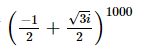Solution:
QUESTION: 8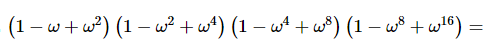Solution:
QUESTION: 9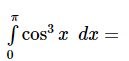Solution:
QUESTION: 10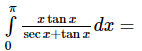Solution:
QUESTION: 11

Let f(x) be a function satisfying f ′ x = f x with f(0) = 1 and g(x) be a function that satisfies f(x) + g(x) = x2, then value of integral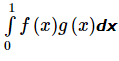is equal to

Solution:
QUESTION: 12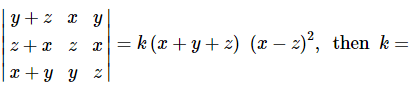Solution:
QUESTION: 13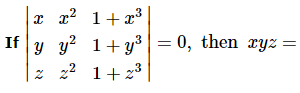Solution:
QUESTION: 14
If x dy = y(dx + y dy), y > 0 and y (1) = 1, then y (-3) is equal to
Solution:
QUESTION: 15
Solution of cos x dy/dx+y sin x =1 is
Solution:
QUESTION: 16
If y = 2ax and $\frac{dy}{dx}$ = log 256 at x = 1, then a =
Solution:
QUESTION: 17
The number of points at which the function f(x)=|x-0.5|+|x-1|+tanx is not differentiable in (0,2) is
Solution:
QUESTION: 18

The eccentricity of the conic 9x2 + 25y2 = 225 is

Solution:
QUESTION: 19

The line y = 4x + c touches the hyperbola x2 - y2 = 1 if

Solution:

We know that the line y = mx + c touches the hyperbola x 2 a 2 - y 2 b 2 = 1, then
c2 = a2m2 - b2
Here the hyperbola is x2 - y2 = 1
ie here a2 = b2 = 1
and comparing y = 4x + c with y = mx + c,
we get
m = 4
∴ c2 = 16 - 1 = 15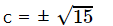QUESTION: 20

The st. line lx + my + n = 0 touches the hyperbola x2/a2 - y22/b2 = 1 if

Solution:
QUESTION: 21
S and T are the foci of an ellipse and B is an end of the minor axis. If STB is an equilateral triangle then the eccentricity of the ellipse is
Solution:
QUESTION: 22

The equation x + ex = 0 has

Solution:

Let f x = x + e x = 0.

Since f − ∞ = − ∞ and f + ∞ = ∞ ,
∴ f x = 0 has a real root.
Let the real root be α . Then f( α ) = 0.
Now , f ′ x = 1 + e x > 0, ∀ x ∈ R
∴ f x is an increasing function ∀ x ∈ R .
∴ for any other real number β ,
f β > f α or f β < f α .
But f a = 0 ; so , f β ≠ 0.
∴ f x = 0 has no other real root.
Hence, the equation has only one real root.

QUESTION: 23

tan⁻1(1/7)+2tan⁻1(1/3)=

Solution:
QUESTION: 24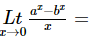Solution:
QUESTION: 25
If A is a singular matrix, then Adj A is
Solution:
QUESTION: 26

The line y=mx+c touches the parabola x2=4ay if

Solution:
QUESTION: 27

If |z₁|=|z₂| and amp. z₁+amp.z₂=0, then

Solution:
QUESTION: 28
The maximum value of x3 - 3x in [0,2] is
Solution:
QUESTION: 29

The normals to the parabola y2=4ax from the point (5a,2a) are

Solution:
QUESTION: 30
The number of five digits telephone numbers having atleast one of their digits repeated is
Solution:
QUESTION: 31

Five digit number divisible by 3 is formed using the digits 0, 1 , 2, 3, 4 and 5 without repetition. Total number of such numbers is

Solution:

A number is divisible by 3 if and only if the sum of its digits are divisible by 3
Notice that 1 + 2 + 3 + 4 + 5 = 15, which is divisible by 3
The only other way we can have a sum of 5 digits divisible by 3 is to replace the 3 by the 0 making the sum 3 less:
1 + 2 + 0 + 4 + 5 = 12, which is divisible by 3
No other choice of 5 digits can have a sum divisible by 3, because there is no other way to make the sum 12 or 15, and we certainly can't have a sum of 9 or 18
So the number of 5-digit numbers that can be formed from the digits {1,2,3,4,5} is

Number of ways = 1 x 2 x 3 x 4 x 5 = 120
And the number of 5-digit numbers that can be formed from the digits {1, 2, 0, 4, 5} is figured this way

Number of ways = 1 x 2 x 3 x 4 x 4 = 96
Total Number of ways = 120 + 96 = 216

QUESTION: 32
The number of 7 digit numbers which can be formed using the digits 1, 2, 3, 2, 3, 3, 4 is
Solution: There are 7 digits 1, 2, 3, 2, 3, 3, 4 in which 2 occurs 2 times and 3 occurs 3 times
Number of 7 digit numbers
= $\frac{7!}{2! 3!}$ = 420
QUESTION: 33
A and B are events such that P(A ∪ B) = 3/4, P(A ∩ B) = 1/4, P(A̅) = 2/3, then P(A̅ ∩ B) is
Solution:
QUESTION: 34
There are 5 volumes of mathematics among 25 books. They are arranged on a shelf in random order. The probability that the volumes of mathematics stand in increasing order from left to right is
Solution:
QUESTION: 35
A card is drawn at random from a pack of cards. The probability of this being red or queen is
Solution:
QUESTION: 36

If two angles of a Δ A B C are 45 º and 60 º then the ratio of the smallest and the greatest sides are

Solution:
QUESTION: 37
If the sides of a triangle are 3,5,7, it has
Solution:
QUESTION: 38

If the equation x3 - ax2 + bx - a = 0 has real roots, then it must be the case that :

Solution:
QUESTION: 39

If α,β are the roots of the equation x2+x+1 = 0 and α/β, β/α are roots of the equation x2+px+q = 0, then p equals

Solution:
QUESTION: 40
If α and β (α < β) be two different real roots of the equation ax2 + bx + c = 0, then
Solution: $\text{Let}\phantom{\rule{0.5em}{0ex}}f\left(x\right)=a{x}^{2}+bx+c.$
$\text{Then},\phantom{\rule{0.5em}{0ex}}f\left(\alpha \right)=0=f\left(\beta \right).$
Also, f(x) is continuous and differentiable in [$\alpha ,\beta$] as it is a polynomial function or x.
Hence, by Rolle's theorem, there exists a k in ($\alpha ,\beta$), such that
$f\prime \left(k\right)=0⇒2ak+b=0⇒k=-\frac{b}{2a}.$
$\therefore \alpha <-\frac{b}{2a}<\beta .$
QUESTION: 41
A G.P. consist of an even number of terms. If the sum of all the terms is 5 times the sum of the terms occupying odd places, then the common ratio will be
Solution:
QUESTION: 42
If (a, b), (c, d), (e, f) are the verices of a triangle such that a, c, e are in G.P. with common ratio r and b, d, f are in G.P. with common ratio's then the area of the triangle is
Solution:
QUESTION: 43
The sum of 10 terms of the series √2 + √6 + √18 +.... is
Solution:
QUESTION: 44
The 6th term of a G.P. is 32 and its 8th term is 128 ; then the common ratio of the G.P. is
Solution: The nth tan of a G.P is (tn) = arn - 1
t6 = a.r5 = 32
t8 = a.r7 = 128
$\frac{{r}^{5}}{{r}^{7}}$ = $\frac{32}{128}$
or $\frac{{1}^{}}{{r}^{2}}$ = $\frac{1}{4}$
or r2 = (2)2
or r = 2
QUESTION: 45
The equation of line passing through lines 5x-6y-1=0 and 3x+2y+5=0 and perpendicular to 3x-5y+11=0 is
Solution:
QUESTION: 46
The equation of line passing through (a,b) and perpendicular to ax+by+c=0 is
Solution:
QUESTION: 47
Set A has 3 elements and set B has 4 elements. The number of injections that can be defined from A to B is
Solution: No. of injections = 4P3 = 24
QUESTION: 48
In a city 20 percent of the population travels by car, 50 percent travels by bus and 10 percent travels by both car and bus. Then persons travelling by car or bus is
Solution:
QUESTION: 49
If $\alpha ,\beta ,\gamma$ are the roots of ${x}^{3}+2{x}^{2}-3x-1=0,\phantom{\rule{0.5em}{0ex}}\text{then}\phantom{\rule{0.5em}{0ex}}{\alpha }^{-2}+{\beta }^{-2}+{\gamma }^{-2}=$
Solution:
QUESTION: 50

If cos2θ=(√2+1)(cosθ-(1/√2)), the value of θ is

Solution:
QUESTION: 51

Let f be real valued function satisfying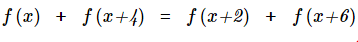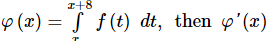and φ x = ∫ x x + 8 f t d t , then φ ′ x is

Solution:
QUESTION: 52

If α , β and γ are the altitudes of the Δ A B C from the vertices A, B and C respectively, then the value of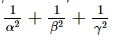is

Solution:
QUESTION: 53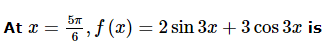Solution:
QUESTION: 54

If f(x) is an even and differentiable function, then the value of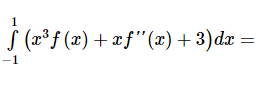Solution:
QUESTION: 55

If the line x cos θ + y sin θ = 2 is the equation of a transverse common tangent to the circles x 2 + y 2 = 16 and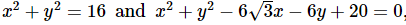, then θ equals

Solution:
QUESTION: 56

If two events A and B are such that P(Ac) = 0.3, P(B) = 0.4 and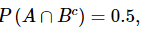, then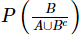is equal to

Solution:
QUESTION: 57

Area bounded by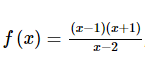, x-axis and ordinates x = 0 and x = 3 2 is

Solution:
QUESTION: 58

The distance between the chords of contact of the tangents to x 2 + y 2 + 2 g x + 2 f y + c = 0 from (0, 0) and (g, f) is

Solution:
QUESTION: 59

Let F denote the set of all onto functions from A = { a 1 , a 2 , a 3 , a 4 } to B = { x , y , z } . A function f is chosen at random from F. The probability that f − 1 consists of exactly one element is

Solution:
QUESTION: 60

For any two complex numbers z1, z2 and a, b ∈ R,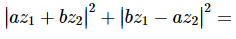Solution:
QUESTION: 61

Let a and b be non-zero real numbers. Then, the equation (ax2 + by2 + c) (x2 - 5xy + 6y2) = 0 represents

Solution:
QUESTION: 62

The values of a, b, c for which the equation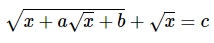has infinitely many solutions are

Solution:
QUESTION: 63

The function defined by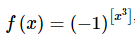, Where [ ] denotes greatest integer function satisfies

Solution:
QUESTION: 64

The number of real roots of the equation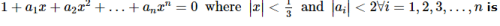Solution:
QUESTION: 65

Tangents drawn from the point P(1,8) to the circle
x 2 + y 2 − 6 x − 4 y − 11 = 0
touch the circle at the points A and B. The equation of the circumcircle of the triangle PAB is

Solution:
*Multiple options can be correct
QUESTION: 66

The equation sin x = [ 1 + sin x ] + [ 1 − cos x ] has
{where [x] is the greatest integer less then or equal to x }

Solution:
*Multiple options can be correct
QUESTION: 67

If n is the number of positive integral solutions of X1 X2 X3 X4 = 210 then

Solution:
*Multiple options can be correct
QUESTION: 68

Let a, b, c, d real numbers. Suppose that all the roots of the equation z4 + az3 + bz2 + cz + d = 0 are complex numbers lying on the circle |z| = 1 in the complex plane. The sum of the reciprocals of the roots is necessarily

Solution:
*Multiple options can be correct
QUESTION: 69

In a certain culture of bacteria, the rate of increase is proportional to the number present. It if be known that the number doubles in 4 hours, then

Solution:
*Multiple options can be correct
QUESTION: 70

Two lines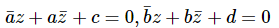0 where a , b ∈ C − { 0 } and c , d ∈ R , are:

Solution:
*Multiple options can be correct
QUESTION: 71

All points lying inside the triangle formed by the points (1, 3), (5, 0) and (-1, 2) satisfy

Solution:
*Multiple options can be correct
QUESTION: 72

Let A(z1), B(z2), C(z3) be the vertices of a triangle in the Argand plane. then which of the following statements is correct?

Solution:
*Multiple options can be correct
QUESTION: 73

Let f x = max { 1 + sin x , 1 , 1 − cos x } , x ∈ [ 0 , 2 π ] and g x = max { 1 , | x − 1 | } ∀ x ∈ R then,

Solution:
*Multiple options can be correct
QUESTION: 74

If P(1, 2), Q(4, 6), R(5, 7) and S(a, b) are the vertices of a parallelogram PQRS, then

Solution:
*Multiple options can be correct
QUESTION: 75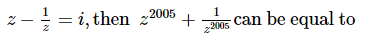Solution:Use Code STAYHOME200 and get INR 200 additional OFF Use Coupon Code